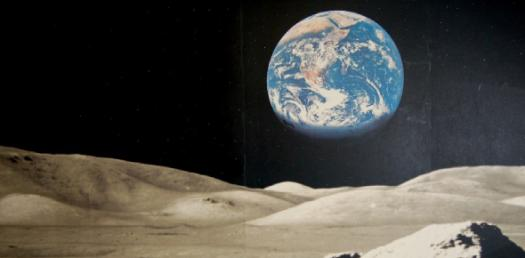# Earth Science: Tectonic Notes

10 Questions | Attempts: 113
ShareSettings• 1.
What is the driving force behind crustal movement?
• A.

The movement in the inner core

• B.

The movement in the outer core

• C.

The movement of convection in the mantle

• 2.
P-waves can travel through......
• A.

Solids

• B.

Liquids

• C.

Gases

• D.

All of the Above

• E.

None of the Above

• 3.
S-waves can travel through....
• A.

Solids

• B.

Liquids

• C.

Gases

• D.

All of the Above

• E.

None of the Above

• 4.
Where are most earthquake epicenters and volcanoes be found?
• A.

In the middle of a plate boundary

• B.

On a plate boundary

• C.

The bottom of a plate boundary

• D.

The top of a plate boundary

• 5.
How do we know that Earth's inner core is solid?
• A.

Because Pwaves refract in the inner core due to that the outer core is liquid

• B.

Because Swaves refract in the inner core due to that the outer core is liquid

• 6.
What is the Richter Scale?
• A.

Based on observations

• B.

Tells the intensity of a volcano

• C.

Tells the intensity of an earthquake

• D.

Based on inferences

• 7.
What type of plate boundary is this?
• A.

Transform

• B.

Divergent

• C.

Convergent

• 8.
What type of plate boundary is this?
• A.

Transform

• B.

Divergent

• C.

Convergent

• 9.
What type of plate boundary is this?
• A.

Transform

• B.

Divergent

• C.

Convergent

• 10.
What provides the energy for convection currents in the Earth's mantle?
• A.

• B.

• C.

Ultraviolet waves

• D.

Gamma waves

## Related TopicsBack to top
×

Wait!
Here's an interesting quiz for you.